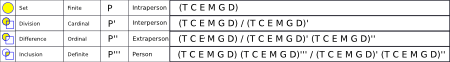## Business Physics III: Energy and Gravity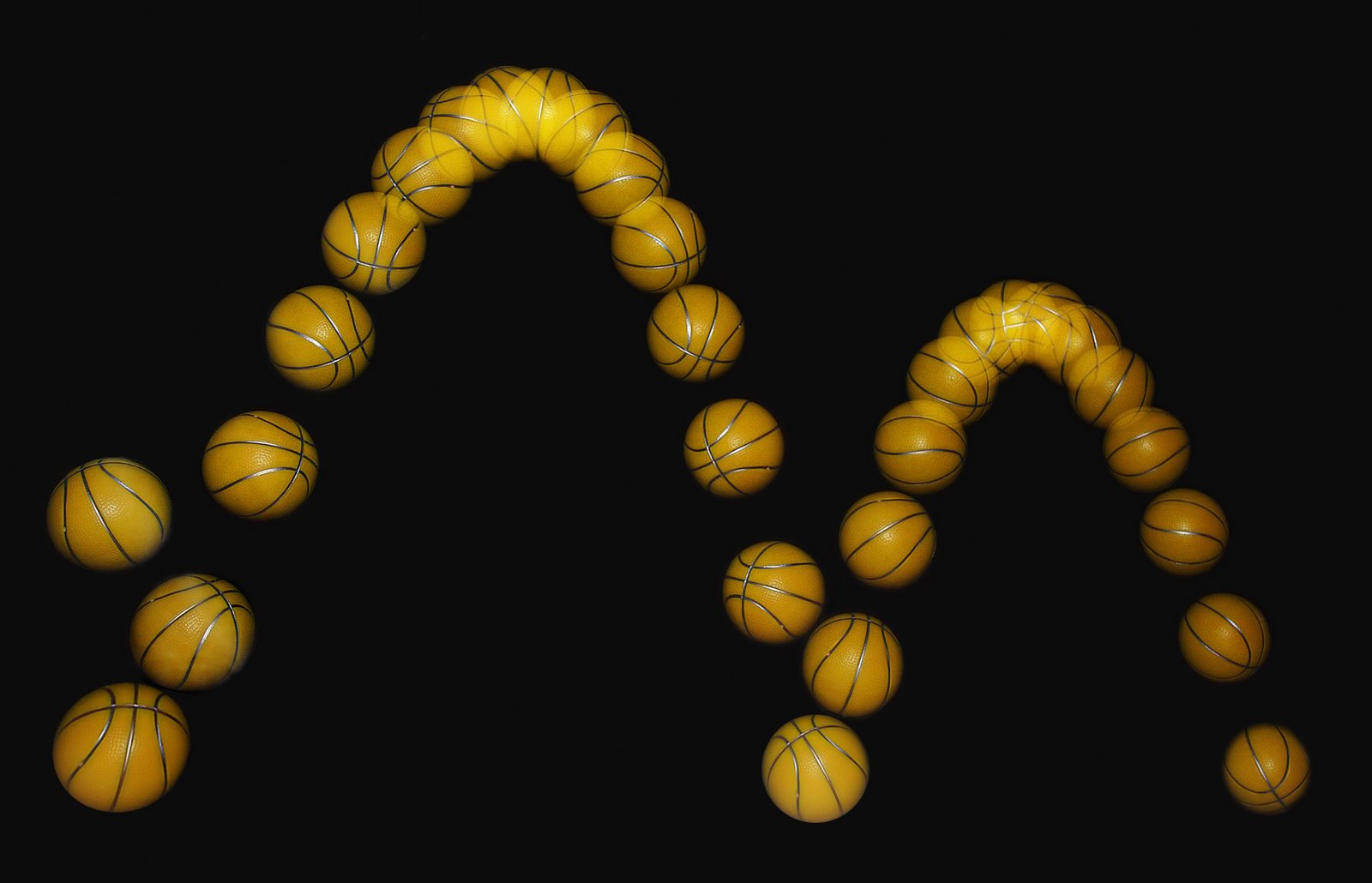In examining an Newton’s and Einstein’s equations an interesting result became apparent to me.  According to Einstein’s equation

E = mc^2

If we consider my four dimensional equations in my previous related post

E = Mass * Light = M * C

or

E = ( ( T * T”’ ) / ( S’ * S” ) ) * ( ( S * S”’ ) / ( T’ * T” ) )

Related Posts:

Business Physics I: Space and Time

Business Physics II: Mass and Light

## Business Physics I: Space and TimeEverything you’ve learned in school as “obvious” becomes less and less obvious as you begin to study the universe. For example, there are no solids in the universe. There’s not even a suggestion of a solid. There are no absolute continuums. There are no surfaces. There are no straight lines.
R. Buckminster Fuller

One of the discoveries I have made, so far, in my study of physics and how it translates to business is that the fundamental building blocks of of the universe are the point and event.

The first step is to create the cardinal Distance set

Distance = | p |

and to create the cardinal Duration set

Duration = | e |

The second step is to create the ordinal Area set

Area = ( p / p’ )

and to create the ordinal Frequency set

Frequency = ( e / e’ )

The third step is to create the exponential Volume set

Volume = ( p / ( p’ * p”)

and the exponential Amplitude set

Amplitude = ( e / ( e’ * e” ) )

Finally, we consider the complete sets of Events giving us Space

Space = ( ( p * p”’ ) / ( p’ * p” ) )

and Time

Time = ( ( e * e”’ ) / ( e’  * e” ) )

where e are event sets and p are point sets.  Space and Time are four dimensional set constructs.

This applies to Business in the sense that Locating (Space) and Timing (Time) are the fundamental building blocks of business:  The first order of business.  We will move up to the next level in the next post.

Related Post:

Business Physics II:  Mass and Light

Business Physics III: Gravity and Energy

## Physics: Within and Without the BoxI have been reading extensively about the thought experiment, called Schrödinger’s Cat, and have reached a personal conclusion that it is inherently flawed by its idealism.  There is no case where any object inside “the box” can be fully shielded from decoherence.  There is no example of this in nature.  Every case for the validity of the experiment is baseless.  Interestingly enough, this is what Schrödinger was out to demonstrate and with which Einstein agreed.  What hadn’t been explored at the time was entanglement can be explained simply by additional dimensions.

Atomic Tunneling

## When – Where## How – What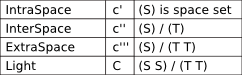## How Much – Why

This is the set of equations I find curious.  As you can see (C)/(C) equals Energy.  Consequently, what are the two higher forms that correlate with Gravity?

## Who – Whom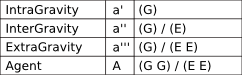## Garrett Lisi on his theory of everything

I agree with Garrett Lisi. It’s sets and geometry.

Vodpod videos no longer available.

more about “Garrett Lisi on his theory of everyth…“, posted with vodpod

The Lie E8 is a beautiful structure mathematically with 248 dimensions: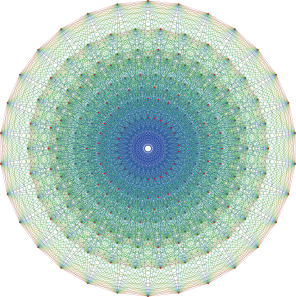However, I think what he wants to achieve is even simpler to model physically.

This is a 3V Icosahedron Geodesate:This structure gives us 92 vertexes (entities) and 270 edges (associations) or 362 particles.

Employing Occam’s Razor, I suggest that some form of Icosahedron Geodesate theory is more likely the answer.

## Universe: Octahedron TheoryThe octahedron:

1. 3 Axes (I thought this was a triangular point)
2. 4 Planar Corners (I thought this was a tetrahedral shell)
3. 6 Vertexes (I thought this was an octahedral shell)
4. 12 Edges (I thought this was an icosahedral shell)

The key is the universe is composed of particles of a broad variety.  But every particle is simply an association in the form of a set.  The lowest order particles are event and point.  They are one dimensional particles.  All subsequent higher dimension particles can be reduced to a subset of these particles.

The three axes of the octahedron are the universes of different orders.  They are simply subsets of one another.

The six vertexes of the octahedron are the vertex dimension sets of the system.

The twelve edges of the octahedron are edge dimension sets between each of the vertex dimension sets.  These edge sets are also particles and the same set equations can be applied to them that were applied to the vertex sets.

To understand the tables you will require high school level physics knowledge and an understanding of basic set theory.

First, I am taking ordinal sets and performing three set operations on them to get subsets.

Second, I am then plugging the subsets into a standard set equation that describes the “space” for that dimension set.

Third, I am then introducing the result into a higher order dimension set.

## When## How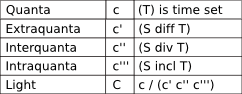## How Much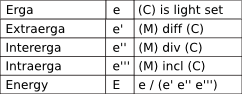## Why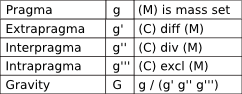## What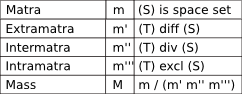## Where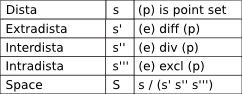## Set Physics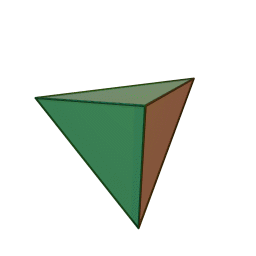I have been giving theoretical physics a thorough and systematic going over and I think I’ve come up with a unified solution that incorporates gravity and all the particles.

I’m thankful for the work of:

• Issac Newton
• Albert Einstein
• R. Buckminster Fuller
• Max Plank
• Richard Feynman
• and a broad array of experimental physicists

Giants whose shoulders I stand on.

Each row in this model is a set of particles.  Everything is ultimately composed of distance and time.

I am creating a new taxonomy because the existing names do not consistently define the particles of the system.

## When## Where## How## What## How Much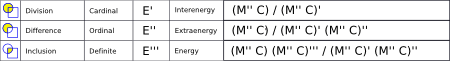## System Schema

The structure of the schema is three dimensional and composed of three shells:

Inner Tetrahedral Intrashell:Middle Octahedral Intershell: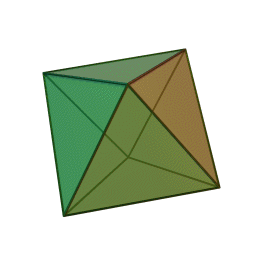Outer Icosahedral Boundary Shell: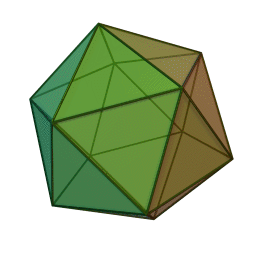The edges, vertexes and connections between the shells are all significant in revealing the dependecy of the particles upon one another.

This is the best I can do for a two dimensional representation: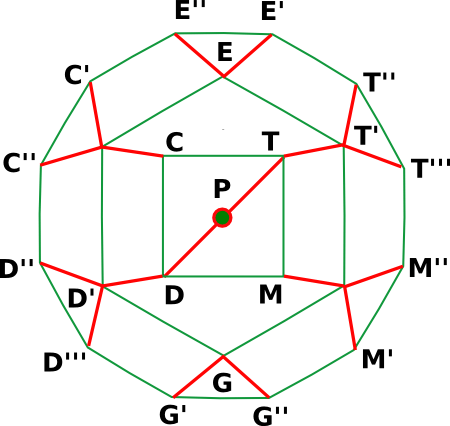## Who